## Abstract

The chemical hydrogen storage properties of ferrosilicon were investigated. A hydrogen yield of ~4.75 wt.% (with respect to the mass of ferrosilicon) was estimated by the reaction of varying quantities of ferrosilicon with 5 mL of 40 wt.% sodium hydroxide solution. The reaction of ferrosilicon with aqueous sodium hydroxide solution to form hydrogen was found to have an activation energy of 90.5 kJ mol−1 by means of an Arrhenius plot. It was observed that the induction period of the hydrogen generation reaction varies exponentially with temperature. Although this combination of high activation energy and a lengthy induction period at low temperatures reduces the attractiveness of ferrosilicon for portable hydrogen storage applications unless methods can be developed to accelerate the onset and rate of hydrogen generation, its low cost and widespread availability make it attractive for further studies focused on higher temperature stationary applications.

## Introduction

In recent years, there has been a great surge of research activity in the area of energy. This is primarily driven by two conflicting factors; the need to satisfy an ever increasing demand for energy while simultaneously reducing greenhouse gas emissions to sustainable levels. Broadly speaking, there are two ways that these goals can be achieved. The first, typically termed carbon capture and storage (CCS), is to capture the emissions from the fuels which provide our energy, and store them in such a way that the levels released to the atmosphere are substantially reduced . This type of technology is well suited to large industrial plants or power stations, but its deployment in vehicular or portable applications would be problematic. The second solution is to switch to using an alternative fuel to hydrocarbons. Hydrogen is arguably the most promising option, given its large amount of chemical energy per unit mass (142 MJ kg−1) and the benign nature of its combustion product, water . However, due to its low density (0.0824 g L−1 at 298 K ), the storage and transportation of hydrogen gas is problematic as it must be heavily compressed. Compressed gases must be stored in heavy steel containers, partially nullifying the benefit in terms of high gravimetric energy density of using hydrogen in the first place. Alternatively, hydrogen can be stored in cryogenic containers, but these are costly and accumulate considerable “boil off” losses . Both of these approaches are also hazardous and present considerable risks to the user and the public at large.

Hence for the deployment of hydrogen in vehicular and portable applications to be viable, alternative storage methods must be developed . A particularly attractive approach is that of chemical hydrogen storage materials, which release their hydrogen when required by means of a chemical reaction [6, 7]. While at first consideration, this may seem a relatively new approach, it has in fact been heavily utilized before. In the first three decades of the 20th century, Zeppelins, huge airships filled with hydrogen, flew regularly across the Atlantic Ocean. They were also deployed in World War I. The filling of the airships required a supply of a substantial volume of hydrogen gas in a form which could be safely stored for months at a time and transported across large distances of uneven, battle torn terrain. To obtain this, the US military made use of a material known as ferrosilicon [8, 9]. Ferrosilicon is an alloy formed of various phases of iron and silicon. It has been used extensively in the steel industry for ~125 years to remove oxygen from melted steel during the casting process, thus improving its physical properties . Ferrosilicon is produced by the reduction of quartz with coke in the presence of iron or iron ore . Ferrosilicon up to 15% silicon content is produced in a blast furnace, while higher silicon content can be reached by the use of a submerged electric arc furnace [10-13]. Each batch has a fixed amount of quartz and a variable amount of coal and iron oxide to attain the desired iron to silicon ratio . The most widely available composition is ferrosilicon 75, which consists of ~75 wt.% silicon, the remaining ~25 wt.% consisting principally of iron (in the form of iron disilicide, FeSi2) with a small amount of aluminium (1–2 wt.%) . To obtain hydrogen for their Zeppelins, the US military reacted ferrosilicon with sodium hydroxide solutions in large batch reactors [8, 9, 15].

It is perhaps surprising that despite the present volume of literature on chemical hydrogen storage materials, there have been no published investigation of the hydrogen generating properties of ferrosilicon since the reports by the US military some three quarters of a century ago. In this article we examine the chemical hydrogen storage properties of ferrosilicon for portable applications.

## Experimental

Ferrosilicon and sodium hydroxide were purchased from Castree Kilns (St. Clears, Carmarthenshire, Wales) and Sigma Aldrich (Gillingham, Dorset, UK), respectively, and used as received. The evolved hydrogen was collected and quantified using the water displacement method [16, 17].

For the evaluation of the volume of hydrogen generated per unit mass of ferrosilicon, 5 mL of 40 wt.% sodium hydroxide solution was added to a 50 mL round bottomed flask in a water bath at 60°C and left for 10 mins to equilibrate. To this was then added the desired mass of ferrosilicon, and the volume of hydrogen evolved recorded over a 60-min period. Each reaction was performed in triplicate.

To determine the activation energy of the reaction, 5 mL of 40 wt.% sodium hydroxide solution was heated in a water bath in a 50 mL round bottomed flask and left to equilibrate for 10 mins. To this was then added 1.00 g of ferrosilicon, and the volume of hydrogen evolved recorded over a 10 min period. Each reaction was performed in triplicate.

## Results and Discussion

### Hydrogen yield

The generation of hydrogen from silicon in alkali solutions is well known and proceeds by the following reaction:[18, 19]

 $2{\mbox{NaOH}}+{\mbox{Si}}+H_{2}O\rightarrow {\mbox{Na}}_{2}{\mbox{SiO}}_{3}+$$2H_{2}$
(1)

In this way, silicon can theoretically produce 14% of its own weight in hydrogen. The literature on ferrosilicon hydrolysis suggests that its hydrogen generation reactions proceed in a similar way [8, 9, 15]. To estimate experimentally the hydrogen yield with respect to the mass of ferrosilicon, 0.75, 1.00, 1.25, and 1.50 g of ferrosilicon were added to a 40 wt.% solution of sodium hydroxide at 60°C, and the hydrogen generation recorded over a period of an hour as shown in Figure 1. After an induction period, hydrogen generation increased rapidly at first before gradually slowing.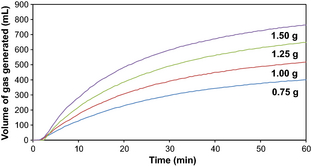Figure 1. A plot of volume of hydrogen generated over time during the reaction of 0.75 g (blue), 1.00 g (red), 1.25 g (green), and 1.50 g (purple) of ferrosilicon with 5 mL of 40 wt.% sodium hydroxide solution in a water bath at 60°C.

As shown in Figure 2, the total volume of hydrogen evolved can be plotted against the mass of ferrosilicon added to the reaction mixture to obtain a linear trend. The total volume of hydrogen evolved can be converted to a mass, and from this the hydrogen yield with respect to the mass of ferrosilicon can be estimated as a reasonably consistent ~4.75 wt.% across these experiments (Fig. 3). This is considerably lower than the theoretical maximum for silicon.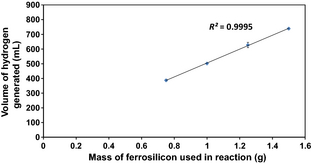Figure 2. A plot of total volume of hydrogen evolved against mass of ferrosilicon used in the reaction.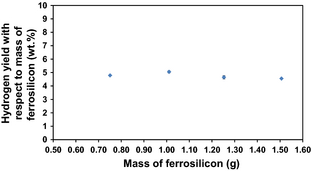Figure 3. A plot of the mass of ferrosilicon used in the reaction against the hydrogen yield with respect to the mass of ferrosilicon.

The order of reaction with respect to ferrosilicon can be obtained from the gradient of a plot of the natural log of the maximum or initial hydrogen generation rate (HGR) against the natural log of the mass of ferrosilicon used in the reaction (Fig. 4), and in this case is estimated to be ~0.7.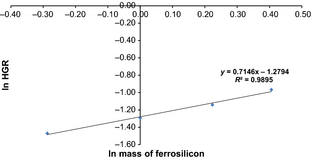Figure 4. A plot of ln HGR against ln mass of ferrosilicon used in each reaction to obtain the order of reaction with respect to ferrosilicon.

### Arrhenius and Eyring plot

To obtain an estimate of the activation energy for the reaction of ferrosilicon with sodium hydroxide solution to form hydrogen, an Arrhenius plot can be constructed [20, 21]. About 1.00 g of ferrosilicon was added to 5 mL of 40 wt.% sodium hydroxide solution in a water bath at 331, 335, 339, 344, and 348 K, and the volume of gas evolved recorded for 10 mins (Fig. 5). This is sufficient to calculate the maximum or initial rate of reaction commonly referred to as the hydrogen generation rate. The Arrhenius equation can be written in the following form:

 $lnk\quad =\quad lnA-(E_{a}/RT)$
(2)

where k is the hydrogen generation rate (mL s−1), A is the pre-exponential factor, Ea is the activation energy (kJ mol−1), R is the gas constant (8.314 J mol−1 K−1) and T is the hydrolysis temperature (K). Hence, the gradient of a plot of ln HGR versus 1/T will give a gradient equal to Ea/R, and from this the activation energy can be determined (Fig. 6). In this case, the activation energy is estimated to be 90.5 kJ mol−1. As can be seen from Table 1, this is substantially higher than the activation energy determined for the hydrolysis reactions of other chemical hydrogen storage materials, such as sodium borohydride and aluminium. We believe that this is due to the presence of iron disilicide in the ferrosilicon matrix, which renders the silicon less available for, and thus slows the rate of, reaction. This sluggish rate of reaction of ferrosilicon is a major disadvantage in the context of portable applications.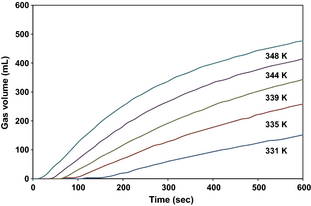Figure 5. Hydrogen generation curves at 331 K (blue), 335 K (red), 339 K (green), 344 K (purple), and 348 K (turquoise) from the reaction of 1.00 g of ferrosilicon with 5 mL of a 40 wt.% sodium hydroxide solution.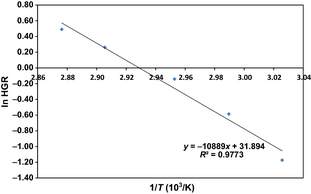Figure 6. Arrhenius plot for the reaction of ferrosilicon with sodium hydroxide solution.

Table 1. Activation energy of various hydrogen generation reactions calculated using the Arrhenius equation
Catalyst Ea (kJ/mol) References
NaBH4
Co–P 23.9 
Co films 27.6 
Ru nanoclusters 28.51 
Co–Ni–P–B 28.7 
Co–W–B 29 
Co–Cu–B 29.9 
Co–Fe–B 31 
Pd–Ni–B 31.1 
Co–B 33 
Co–Ni–B 34 
Co–Cr–B 37 
Co–Ni–P 38 
Co–PVP 38.7 

Hydrogen generating mixture Ea (kJ/mol) References
Al based systems
Al–Bi–Li–NaCl 22.57 
Al–Li–In–Zn 22.91 
Al–Li3AlH6 33 
Al–Ga–In–Sn 43.8 
Al powder 64.2 
Activated aluminium 69 
Al/NaAlO2 71 

Further information about the reaction kinetics can be obtained from an Eyring plot [20, 21]. The Eyring equation can be expressed as follows:

 $lnk/T\quad =\quad -\Delta H^{\not =}/R.1/T+ln\left(k_{b}/h\right)+$$\Delta S^{\not =}/R$
(3)

where k is the hydrogen generation rate (mL s−1), R is the gas constant (8.314 J mol−1 K−1), T is the hydrolysis temperature (K), kb is the Boltzmann constant, h is the Planck constant, and ΔH and ΔS are the activation enthalpy and entropy of the hydrolysis reaction, respectively. Thus, a plot of ln k/T against 1/T yields a straight line graph with a gradient of ΔH/R and a y axis intercept of ln (kb/h) + ΔS/R. This is presented in Figure 7, and gives a value for the activation enthalpy of 87.7 kJ mol−1 and a value for the activation entropy of 10.9 J K−1 mol−1. The sign of the activation entropy gives an indication of whether the rate determining step is an associative (negative value) or dissociative (positive value) step. Previous studies on the etching of bulk silicon in hydroxide solutions show that that reaction proceeds by the following steps:[18, 38]

1. Hydroxide attack of bulk silicon
 ${\mbox{Si}}+2{\mbox{OH}}^{-}+4h^{+}\rightarrow {\mbox{Si(OH)}}_{2}^{2+}$
2. Reduction of water
 $4H_{2}O\rightarrow 4{\mbox{OH}}^{-}+2H_{2}+4h^{+}$
3. Formation of a water-soluble complex
 ${\mbox{Si(OH)}}_{2}^{2+}+4{\mbox{OH}}^{-}\rightarrow {\mbox{SiO}}_{2}{\mbox{(OH)}}_{2}^{2-}+$$2H_{2}O$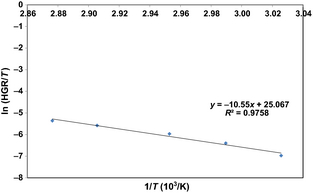Figure 7. Eyring plot for the reaction of ferrosilicon with sodium hydroxide solution.

Step (1) was found to be the rate determining step, and, as it is an associative step, a negative activation entropy is expected for this reaction. As we obtained a positive activation entropy, our results suggest that the etching of silicon in ferrosilicon powders follows a slightly different mechanism to the etching of bulk silicon, wherein a dissociative step, possibly the separation of silicon from the ferrosilicon matrix, is rate determining. However, given the closeness to zero of the activation entropy and the uncertainty in the trend line, it is difficult to be certain.

The onset of hydrogen evolution is not instant. The time required for the onset of hydrogen evolution is found to vary exponentially with temperature (Fig. 8). In the reaction of silicon with sodium hydroxide solutions, a similar induction period is observed, which is typically ascribed to the etching of the surface silicon dioxide layer which must be accomplished before hydrogen generation can commence . This has been found to be an Arrhenius process, and it seems likely that the induction period for ferrosilicon is caused by this. Extrapolation of the trend line from Figure 8 towards room temperature indicates that ferrosilicon is unsuitable as a hydrogen storage material for low temperature portable applications as, notwithstanding the slow kinetics of the reaction once it had commenced, the delay period before the onset of hydrogen production would be much too long for practical application.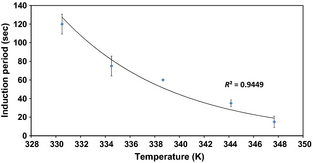Figure 8. The variation in induction period with temperature for the reaction of 1.00 g of ferrosilicon with 5 mL of 40 wt.% sodium hydroxide solution in a water bath.

## Conclusions

Ferrosilicon is cheap, commercially available and safe to transport and handle. However, reactions of ferrosilicon with solutions of sodium hydroxide are dogged by slow kinetics (Ea = 90.5 kJ mol−1) and long induction periods at low temperatures. This suggests that although ferrosilicon is potentially useful for higher temperature, for example, stationary, applications, its utility as a chemical hydrogen storage material for portable applications is limited unless methods can be found to accelerate the onset and rate of hydrogen generation.

## Acknowledgements

The authors thank the EPSRC and Intelligent Energy Ltd for funding this project. PB also thanks the SCI for the award of a Messel Scholarship.

### Document informationPublished on 01/06/17
Submitted on 01/06/17

Licence: Other

### Document Score0

Views 271
Recommendations 0